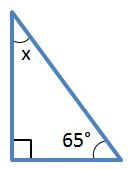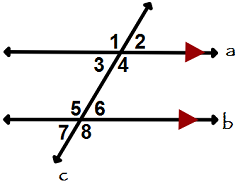# OGET Oklahoma General Education Test (CEOE) (174): Practice & Study Guide Final Exam

Free Practice Test Instructions:

Choose your answer to the question and click 'Continue' to see how you did. Then click 'Next Question' to answer the next question. When you have completed the free practice test, click 'View Results' to see your results. Good luck!

#### Question 3 3. In the right triangle pictured below, what is the value of angle x?#### Question 12 12. Consider the figure below, where Angle 4 = 12m - 4 Angle 5 = 10m + 20 Calculate the measure of Angle 5.